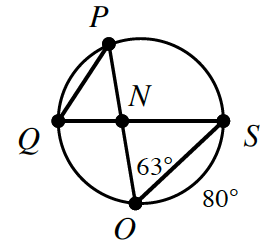### Home > CCG > Chapter 10 > Lesson 10.1.4 > Problem10-43

10-43.

If $\overline{QS}$ is a diameter and $\overline{PO}$ is a chord of the circle at right, find the measure of the geometric parts listed below.1. $m∠QSO$

$\overarc{QO} + \overarc{OS} = \overarc{QS}$

$\overarc{OS}=80°$ and $\overarc{QS}=180°$

$\overarc{QO}+80°=180°$

$\overarc{QO}=100°$

An intercepted arc is twice its inscribed angle.

$m∠QSO=50°$

1. $m∠QPO$

An intercepted arc is twice its inscribed angle.

$50°$

1. $m∠ONS$

$67°$

1. $m\overarc{ P S }$

An intercepted arc is twice its inscribed angle.

$126°$

1. $m\overarc{ P Q }$

$54°$

1. $m∠PQN$

$63°$